# GSEB Solutions Class 12 Chemistry Chapter 8 d-and f-Block Elements

Gujarat Board GSEB Textbook Solutions Class 12 Chemistry Chapter 8 d-and f-Block Elements Textbook Questions and Answers, Additional Important Questions, Notes Pdf.

## Gujarat Board Textbook Solutions Class 12 Chemistry Chapter 8 d-and f-Block Elements

### GSEB Class 12 Chemistry d-and f-Block Elements InText Questions and Answers

Question 1.
Silver atom has completely filled d orbitals (4d10) in its ground state. How can you say that it is a transition element?
Silver exhibits a +2 oxidation state. In this state, the 4d- sub-shell contains nine electrons i.e., one of the 4d-orbitals is partially filled. Hence, it can be regarded as a transition element.Question 2.
In the series Sc (Z = 21) to Zn (Z = 30), the enthalpy of atomisation of zinc is the lowest, i.e., 126 kJ mol-1. Why?
In the formation of metallic bonds, no electrons from 3d-orbitals are involved in case of zinc, while in all other metals of the 3d series, electrons from the d-orbitals are always involved in the formation of metallic bonds.

Question 3.
Which of the 3d series of the transition metals exhibits the largest number of oxidation states and why?
Mn (Z = 25) exhibits the largest number of oxidation state, because it has the maximum number of unpaired electrons. Hence, it shows oxidation states from +2 to +7.

Question 4.
The E°(M2+/M) value for copper is positive (+ 0.34V). What is possibly the reason for this? (Hint: consider its high ∆aH° and low ∆hydH°)
The high energy to transform Cu(s) to Cu2+(aq) is not balanced by its hydration enthalpy.

Question 5.
How would you account for the irregular variation of ionisation enthalpies (first and second) in the first series of the transition elements?
Irregular variation of ionisation enthalpies (first and second) is mainly due to varying degree of stability of different 3d-configurations. d0, d5 and d10 configurations have extra stability and hence in such cases the value of ionisation enthalpies are usually high.

For example, Cr has low first ionisation enthalpy because the electron has to be removed from 4s-orbital but the second ionisation enthalpy is very high as Cr+ has a stable d5 configuration. Zn has very high first ionisation enthalpy because electron is to be removed from stable configuration 3d10 4s2.Question 6.
Why is the highest oxidation state of a metal exhibited in its oxide or fluoride only?
Because of its small size and high electronegativity oxygen or fluorine can oxidize the metal to its highest oxidation state.

Question 7.
Which is a stronger reducing agent Cr2+ or Fe2+ and why?
Cr+2 is a stronger reducing agent than Fe+2. This is because the configuration of Cr+2 changes from d4 to d3 and the d3 configuration is stable (t32g) being half-filled t2g level.

Question 8.
Calculate the ‘spin only’ magnetic moment of Mg2+(aq) ion (Z = 27)?
The divalent ion with atomic number 27 will have d7 configuration.
∴ µ = $$\sqrt{n(n+2)}$$ BM = $$\sqrt{3(5)}$$ = 3.87 BM

Question 9.
Explain why Cu2+ ion is not stable in aqueous solutions?
Cu+(aq) is not stable in an aqueous solution because of its less negative enthalpy of hydration than Cu+2(aq).Question 10.
Actinoid contraction is greater from element to element than lanthanoid contraction. Why?
The 5f electrons are more effectively shielded from the nuclear charge. In other words, the 5f electrons themselves provide poor shielding from element to element in the series.

### GSEB Class 12 Chemistry d-and f-Block Elements Text Book Questions and Answers

Question 1.
Write down the electronic configuration of:

1. Cr3+
2. Pm3+
3. Cu+
4. Ce4+
5. CO2+
6. Lu2+
7. Mn2+
8. Th4+

1. Cr3+ – [Ar] 3d3
2. Pm3+ – [Xe] 4f55d5
3. Cu+ – [Ar] 3d10
4. Ce4+ – [Xe] 4f°
5. CO2+ – [Ar] 3d7
6. Lu2+ – [Xe] 4f14 5d1
7. Mn2+ – [Ar] 3d5
8. Th4+ – [Rn] 5f1Question 2.
Why are Mn2+ compounds more stable than Fe2+ towards oxidation to their +3 state?
It is because Mn2+ has a 3d5 configuration which has extra stability.

Question 3.
To what extent do the electronic configurations decide the stability of oxidation states in the first series of the transition elements? Illustrate your answer with examples?
For the first five elements in the first transition series, the minimum oxidation state is equal to the number of electrons in the outermost s-subshell and the maximum oxidation state is equal to the number of ‘s’ and ‘d’ electrons.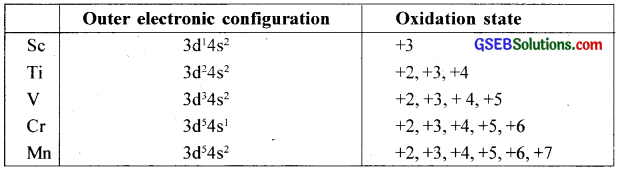Question 4.
What may be the stable oxidation state of the transition elements with the following d electron configurations in the ground state of their atoms: 3d3, 3d5, 3d8 and 3d4?
Stable oxidation states.

+2, +3, + 4, and +5

3d5 (Chromium):
+3, +4, +6

3d5 (Manganese):
+2, +4, +6, +7

3d8 (Cobalt):
+2, +3 (in complexes)

3d4:
There is no d4 configuration in the ground state.Question 5.
Name the oxometal anions of the first series of the transition metals in which the metal exhibits the oxidation state equal to its group number?
Cr2O72- and CrO42- (Group No. = Oxidation state of Cr = 6), MnO4 (Group No. = Oxidation state of Mn = 7).

Question 6.
What is lanthanoid contraction? What are the consequences of lanthanoid contraction?
The steady decrease in the size of the atoms or ions from lanthanum to lutetium is known as lanthanide contraction. As the atomic number increases in the lanthanide series, the new electron goes to the inner 4f orbitals. The shielding effect of a 4f electron is poor.

As a result, the attraction of the outer electrons by the nucleus increases and this tends to a decrease in size. Due to lanthanide contraction, the lanthanides have similar atomic and ionic sizes. Thus, they occur together and the separation becomes very difficult.

Question 7.
What are the characteristics of the transition elements and why are they called transition elements? Which of the d-block elements may not be regarded as the transition elements?
Transition elements are those elements in which the atoms or ions (in stable oxidation state) contain partially filled cf-orbital. These elements lie in the p-block and show a transition of properties between 5-block and p-block. Therefore, these are called transition elements. Elements such as Zn, Cd, and Hg cannot be classified as transition elements because these have completely filled d-subshell. The electronic configurations of the three transition series are given in the table.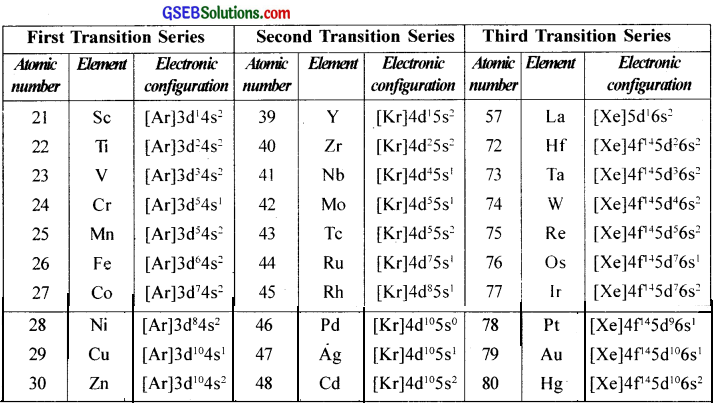The transition elements are those elements which have partially filled d-subshells in their elementary form or in any one of their oxidation states.

This definition of transition elements does not cover zinc, cadmium and mercury because they have completely filled d-subshell in their atoms and common oxidation states. As a result, Zn, Cd, and Hg do not exhibit characteristic properties of transition elements. However, they are studied along with the d-block elements. (Zn, Cd, and Hg show some resemblance to the transition elements and hence are called pseudo-transition elements).Question 8.
In what way is the electronic configuration of the transition elements different from that of the non-transition elements?
In non-transition elements (s and p block), the valence electrons are present only in the outermost shell. But in transition elements, the valence electrons are present in the outermost shell and in the penultimate d subshell.

Question 9.
What are the different oxidation states exhibited by the lanthanoids?
The electronic configurations of the three transition series are given in the table.

Question 10.
Explain giving reasons?

1. Transition metals and many of their compounds show paramagnetic behaviour.
2. The enthalpies of atomisation of the transition metals are high.
3. The transition metals generally form coloured compounds.
4. Transition metals and their many compounds act as good catalysts.

1. Transition metals have unpaired electrons and hence they show paramagnetic behaviour.
2. Due to the presence of unpaired electrons in the valence shell of transition metals, there is strong interatomic bonding in them and hence have high enthalpies of atomisation.
3. In transition metals, there are unpaired electrons and these electrons can readily undergo d-d transition and hence they form coloured compounds.
4. Transition metals have vacant orbitals and can show variable oxidation states. Therefore they act as good catalysts.

Question 11.
What are interstitial compounds? Why are such compounds well known for transition metals?
Most of the Transition elements form interstitial compounds at high temperatures with atoms of non-metallic elements like-H, B, C, N, Si, etc. Small atoms of these non-metallic elements fit in the interstitial voids of the crystal lattice of transition elements. These are called interstitial compounds.

Properties:

1. Properties of these compounds are like parent metal but physical properties differ.
2. These compounds are hard.
3. Their ductility and malleability become less.
4. Their electrical conductivity decreases.
5. Example: TiH, NiH2.Question 12.
How is the variability in oxidation states of transition metals different from that of non-transition metals? Illustrate with examples?
In transition elements, the successive oxidation states differ by unity. For example, Mn shows all the oxidation states from +2 to +7. On the other hand, non-transition metals exhibit variable oxidation states which differ by two units. For example Pb(II), Pb(IV), Sn(II), Sn(IV).

Question 13.
Describe the preparation of potassium dichromate from iron chromite ore. What is the effect of increasing pH on a solution of potassium dichromate?
Potassium dichromate (K2Cr2O7)

Preparation:
On heating chromite ore (FeCr2O4) with sodium carbonate in the presence of air gives sodium chromate.
4FeCr2O4 + 8Na2CO3 + 7O2 → 8Na2CrO4 + 2Fe2O3 + 8CO2

The soluble sodium chromate (Na2CrO4) on acidification with sulphuric acid produces sodium dichromate (Na2Cr2O7) which on treatment with potassium chloride gives crystals of potassium dichromate.

Potassium dichromate is an orange-red crystalline substance. On heating, it decomposes to potassium chromate, chromium oxide, and oxygen.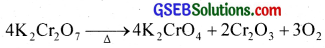Structure:
The chromate ion (CrO42-) and dichromate ion (Cr2O72-) exist in equilibrium and are interconvertible by altering the PH of the solution.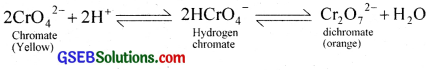The structure of the dichromate and chromate ions are shown in the figure.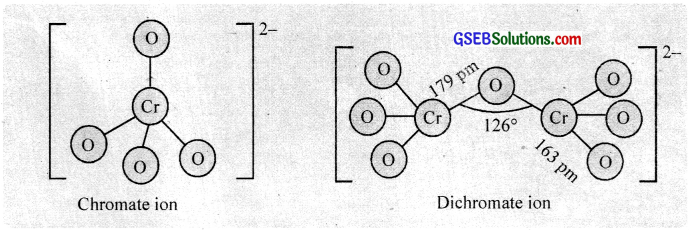In alkaline solution. the dichromate ions are converted to chromate ions; acidification results in the formation of dichromate ions. The four oxygen atoms around the chromium atom are oriented in a tetrahedral arrangement.

Oxidising properties:
Potassium dichromate is a powerful oxidising agent. In an acidic solution, its oxidising action can the represented as follows.
Cr2O72- + 14H+ + 6e → 2Cr3+ + 7H2O [E° = +1.33 V]
K2Cr2O7 + 4H2SO4 → K2SO4 + Cr2(SO4)3 + 4H2O + 3[O]

(a) It oxidises iodides to iodine.
K2Cr2O7 + 7H2SO4 + 6KI → 4K2SO4 + Cr2(SO4)3 + 3I2 + 7H2O
Cr2O72- + 14H+ + 6I → 2Cr3+ + 7H2O + 3I2
This reaction is used in the estimation of iodide ions in volumetric analysis.

(b) It oxidises H2S to S.
K2Cr2O7 + 4H2SO4 + 3H2S → K2SO4 + Cr2(SO4)3 + 7H2O + 3S
Cr2O72- + 8H+ + 3H2S → 2Cr3+ + 7H2O + 3S

(c) It oxidises ferrous salts to ferric salts.
K2Cr2O7 + 7H2SO4 + 6FeSO4 → K2SO4 + Cr2(SO4)3 + 3Fe2(SO4)3 + 7H2O
Cr2O72- + 14H+ + 6Fe2+ → 2Cr3+ + 6Fe3+ + 7H2O
This reaction is used in the estimation of ferrous ions in volumetric analysis.

(d) It oxidises Sn2+ ions to Sn4+ ion
3Sn2+ + Cr2O7-2 + 14H+ → 3Sn4+ + 2Cr3+ + 7H2O

Uses:

• It is used as a primary standard in volumetric analysis.
• In inorganic chemistry, it is used as an oxidising agent.
• In industry, it is used in chrome tanning. preparation of chrome alum, in calico printing, dyeing, etc.

In an aqueous solution, the dichromate and chromate ions are in equilibrium. On increasing the ρH of the solution by adding alkali, the orange-colored dichromate ions are converted into yellow coloured chromate ions.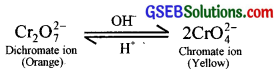Question 14.
Describe the oxidising action of potassium dichromate and write the ionic equations for its reaction with:

1. Iodide
2. Iron(II) solution and
3. H2S

Potassium dichromate (K2Cr2O7)

Preparation:
On heating chromite ore (FeCr2O4) with sodium carbonate in the presence of air gives sodium chromate.
4FeCr2O4 + 8Na2CO3 + 7O2 → 8Na2CrO4 + 2Fe2O3 + 8CO2

The soluble sodium chromate (Na2CrO4) on acidification with sulphuric acid produces sodium dichromate (Na2Cr2O7) which on treatment with potassium chloride gives crystals of potassium dichromate.

Potassium dichromate is an orange-red crystalline substance. On heating, it decomposes to potassium chromate, chromium oxide, and oxygen.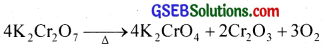Structure:
The chromate ion (CrO42-) and dichromate ion (Cr2O72-) exist in equilibrium and are interconvertible by altering the PH of the solution.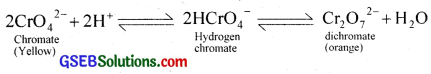The structure of the dichromate and chromate ions are shown in the figure.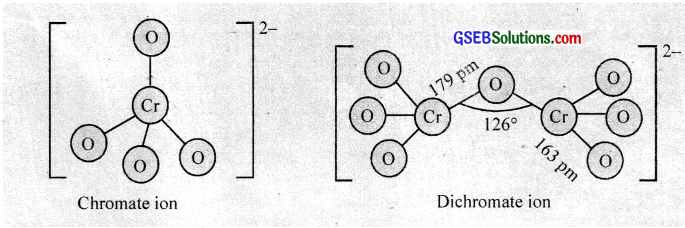In alkaline solution. the dichromate ions are converted to chromate ions; acidification results in the formation of dichromate ions. The four oxygen atoms around the chromium atom are oriented in a tetrahedral arrangement.

Oxidising properties:
Potassium dichromate is a powerful oxidising agent. In an acidic solution, its oxidising action can be represented as follows.
Cr2O72- + 14H+ + 6e → 2Cr3+ + 7H2O [E° = +1.33 V]
K2Cr2O7 + 4H2SO4 → K2SO4 + Cr2(SO4)3 + 4H2O + 3[O]

(a) It oxidises iodides to iodine.
K2Cr2O7 + 7H2SO4 + 6KI → 4K2SO4 + Cr2(SO4)3 + 3I2 + 7H2O
Cr2O72- + 14H+ + 6I → 2Cr3+ + 7H2O + 3I2
This reaction is used in the estimation of iodide ions in volumetric analysis.

(b) It oxidises H2S to S.
K2Cr2O7 + 4H2SO4 + 3H2S → K2SO4 + Cr2(SO4)3 + 7H2O + 3S
Cr2O72- + 8H+ + 3H2S → 2Cr3+ + 7H2O + 3S

(c) It oxidises ferrous salts to ferric salts.
K2Cr2O7 + 7H2SO4 + 6FeSO4 → K2SO4 + Cr2(SO4)3 + 3Fe2(SO4)3 + 7H2O
Cr2O72- + 14H+ + 6Fe2+ → 2Cr3+ + 6Fe3+ + 7H2O
This reaction is used in the estimation of ferrous ions in volumetric analysis.

(d) It oxidises Sn2+ ions to Sn4+ ion
3Sn2+ + Cr2O7-2 + 14H+ → 3Sn4+ + 2Cr3+ + 7H2O

Uses:

1. It is used as a primary standard in volumetric analysis.
2. In inorganic chemistry, it is used as an oxidising agent.
3. In industry, it is used in chrome tanning. preparation of chrome alum, in calico printing, dyeing, etc.
• Cr2O72- + 14H+ + 6I → 2Cr3+ + 7H2O + 3I2
• Cr2O72- + 14H+ + 6Fe2+ → 2Cr3+ + 7H2O + 6Fe3+
• Cr2O72- + 14H+ + 3H2S → 2Cr3+ + 7H2O + 3S

Question 15.
Describe the preparation of potassium permanganate. How does the acidified permanganate solution react with

1. Iron(II) ions
2. SO2 and
3. Oxalic acid? Write the ionic equations for the reactions.

Preparation:
On fusing pyrolusite ore (MnO2) with KOH in the presence of atmospheric oxygen or an oxidising agent like KNO3 or KClO3, a green mass of K2MnO4 is obtained. It is extracted with water and is oxidised to purple coloured potassium permanganate. either electrolytically or bypassing chlorine or ozone into the solution. The purple coloured solution obtained is concentrated by evaporation which on cooling gives crystals of KMnO4.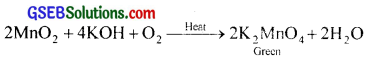MnO42- → MnO4 + le
2K2MnO4 + Cl2 → 2KMnO4 + 2KOH + O2

Potassium permanganate is a purple coloured crystalline solid which on strong heating gives oxygen.
2KMnO4 → K2MnO4 + MnO2 + O2

Structure:
Manganese in MnO4 is in the state of sp hybridisation and hence four oxygen atoms are arranged tetrahedrally around manganese.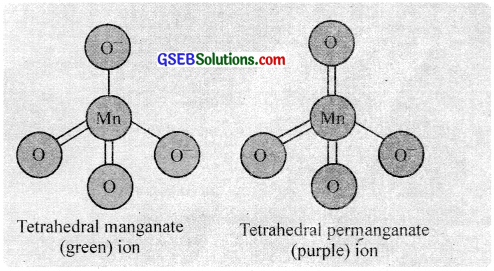Oxidising properties:
KMnO4 is a strong oxidising agent. The oxidation power depends on the use of the permanganate in (a) acidic, (b) neutral or (c) alkaline medium.

(a) In acidic medium:
2KMnO4 + 3H2SO4 → K2SO4 + 2MnSO4 + 3H2O + 5[O]

(b) In neutral medium:
2KMnO4 + H2O → 2KOH + 2MnO2 + 3[O]

(c) In alkaline medium:
2KMnO4 + 2KOH → 2K2MnO4 + H2O + [O]

(a) In acidic medium potassium pcrrnanganatc oxidises

(i) Ferrous salt (green) to ferric salt (yellow).
MnO4 + 8H+ + 5Fe2+ → Mn2+ + 4H2O + 5Fe3+
2KMnO4 + 8H2SO4 + 10FeSO4 → K2SO4 + 2MnSO4 + 5H2(SO4)3 + 8H2O

(ii) Oxalates to carbondioxide.
2MnO4- + 16H+ + 5C2O42- → Mn2+ + 8H2O + 10CO2

(iii) Iodides to iodine:
2MnO4 + 16H+ + 10I → 2Mn2+ + 8H2O + 5I2

(iv) H2 to sulphur:
2KMnO4 + 3H2SO4 + 5 H2S → K2SO4 + 2MnSO4 + 8H2O + 5S

(v) Sulphur dioxide to sulphates:
2KMnO4 + 5SO2 + 2H2O → K2SO4 + 2MnSO4 + 2H2SO4

(b) In neutral or faintly alkaline solution potassium permanganate oxidises

(i) iodides to iodates.
KI + 2KMnO4 + H2O → KlO3 → 2MnO2 + 2KOH

(ii) thiosuiphate to sulphate
8MnO4 + 3S2O32- + H2O → 8MnO2 + 6SO2-4 + 2OH

(iii) manganous salt to MnO2
2MnO4 + 3Mn2+ + 2H2O → 5MnO2 + 4H+
The presence of zinc sulphate or zinc oxide catalyses the oxidation.

Permanganate titrations in presence of HCl are not satisfactory because HCl is oxidised to Cl2.

Uses:
KMnO4 is used

• As an oxidising agent in laboratory and industry.
• In volumetric estimation of ferrous salts, oxalates and other reducing agents.
• As disinfectant in water.
• For qualitative detection of halides, oxalates, tartarates etc.
• In organic reactions under the name Baeyer’s reagent which is alkaline KMnO4.
• In dry cells.Question 16.
For M2+/M and M3+/M2+ systems, the E° values for some metals are as follows: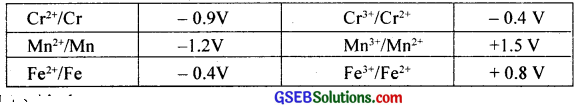Use this data to comment upon

1. The stability of Fe3+ in acid solution as compared to that of Cr3+ or Mn3+ and
2. The ease with which iron can be oxidised as compared to a similar process for either chromium or manganese metal.

1. Cr3+/ Cr2+ has a negative reduction potential. Hence Cr3+ cannot be reduced to Cr2+ i.e., Cr3+ is most stable. But Mn3+/ Mn2+ has larger positive E° value and hence Mn3+ can be easily reduced to Mn2+ i.e., Mn3+ is least stable.
The E° value of Fe3+/ Fe2+ is positive but small and hence Fe3+ is more stable than Mn3+ and less stable than Cr3+.

2. Mn > Cr > Fe. As the E° values of Mn2+/ Mn decrease, the oxidising power also decreases.

Question 17.
Predict which of the following will be coloured in aqueous solution?
Ti3+, V3+, Cu+, Sc3+, Mn2+, Fe3+ and Co2+. Give reasons for each?
Except for Sc3+, all others will be coloured in aqueous solution because incompletely filled 3d – orbitals will give rise to d – d transitions.

Question 18.
Compare the stability of the +2 oxidation state for the elements of the first transition series.
Variable valency is one of the most striking features of the transition elements. All transition elements, except the first and the last members of each series exhibit variable oxidation states.

The cause of showing different oxidation states is because of the small difference between the energies of electrons in the ‘ns’ and ‘(n – 1)d’ orbitals. Thus both ‘ns and ‘(n – 1)d’ electrons can be used for compound formation. i.e., the variable oxidation states of transition elements are related to their electronic configurations.

Let us illustrate the relation between the outer electronic configuration of a transition element and its various possible oxidation slates by taking the example of Mn (3d5 4s2) which shows oxidation states from +2 to +7.

• Formation of Mn2+ (e.g. MnO) involves only two 4s electrons.
• Formation of Mn3+ (e.g. Mn2O3) involves two 4s and one 3d electron.
• Formation of Mn4+ (e.g. MnO2) involves two 4s and two 3d electrons.
• Formation of Mn5+ (e.g. Mn2O5) involves two 4s and three 3d electrons.
• Formation of Mn6+ (e.g. K2MnO4) involves two 4s and four 3d electrons.
• Formation of Mn7+ (e.g. KMnO4) involves two 4s and five 3d electrons.

The multiple oxidation states of the transition elements are due to the availability of d electrons for bond formation. The highest oxidation state shown by any transition metal is +8. The various oxidation states of the elements in the first transition series are shown in the table.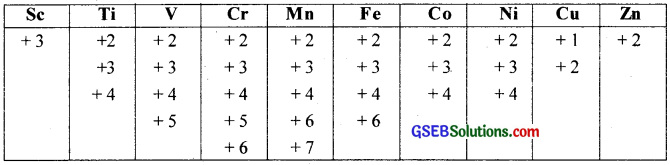A study of the common oxidation states reveals the following.

(i) The most common oxidation state of the first row elements is +2 except in the case of scandium (+3). This is due to the loss of two 4s electrons and one 3d electron. This implies that after scandium, d-orbitals become more stable than s-orbitals.

(ii) The bonds formed by metals in the +2 and +3 oxidation states are mostly ionic. In compounds formed with fluorine or oxygen where the metal exhibiting higher oxidation states, the bonds formed arc essentially covalent. All the bonds formed between manganese and oxygen in the permanganate. MnO4 are covalent.

(iii) Within the same group, the maximum oxidation number increases with atomic number. For example, iron shows the common oxidation state of +2 and +3 but ruthenium and osmium in the same group form compounds +4, +6, and +8 oxidation states.

(iv) Low oxidation states such as +1 and 0 are also found in some transition elements. The common examples are [Ni(CO)4], [Fe(CO)5] in which nickel and iron are in zero oxidation state.Question 19.
Compare the chemistry of actinoids with that of the lanthanoids with special reference to:

1. Electronic configuration
2. Atomic and ionic sizes
3. Oxidation state
4. Chemical reactivity.

Both lanthanide and actinide elements involve the filling of f – orbitals. They show close resemblances in some of their properties, but also differ in many properties.

Similarities:

1. Both exhibit a +3 oxidation state predominantly.
2. In both series, f – orbitals are being progressively filled.
3. Both are electropositive and very reactive.
4. Both exhibit magnetic and spectral properties.
5. Like lanthanide contraction, actinide shows actinide contraction due to the poor shielding effect of 5f electrons.
6. The lanthanide ions other than f° (La3+ or Ce4+) and f14 (Yb2+ and Lu3+) show paramagnetism due to the presence of unpaired f electrons. Neodymium shows maximum paramagnetism.

Differences:
The important points of difference between lanthanides and actinides are summed up below.

 Lanthanides Actinides 1. Besides the common oxidation state of+3, lanthanides show +2 and +4 states. 1. Besides the common oxidation state of+3, actinides show higher oxidation states of +4, +5, +6, and +7. 2. The tendency to form complexes is less 2. They have a greater tendency to form complexes. 3. Except for promethium, all elements are non-radioactive. 3. All actinides are radioactive. 4. Lanthanide compounds are less basic. 4. Actinide compounds are more basic.

In period 7 after Z = 89, when the 5f and 6d-orbitals have almost the same energy. there are 14 actinides which are formed when the 5f-orbitals are filled successively with electrons. There is no 5f electron in thorium. Occupation of electrons in 5f orbitals starts from Pa.

(i) Electronic configuration of Actinide elements: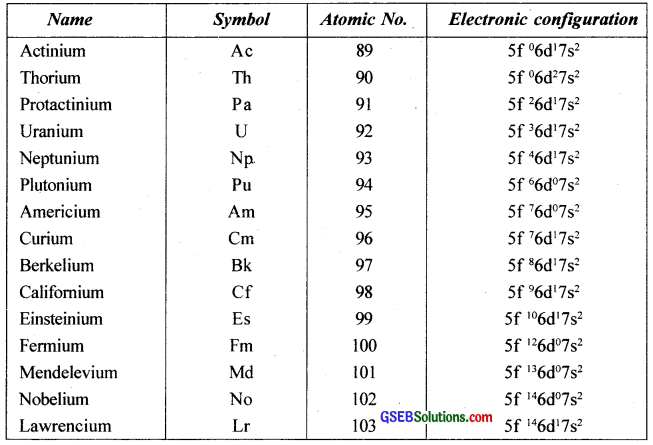(ii) There is a decrease in the size of atoms and ions with an increase in atomic number and is termed as actinide contraction. The decrease is more than in lanthanides because the shielding by 5f is less effective than that by 4f electrons.

Other properties:
Actinides are more reactive than lanthanides. This is partly due to the bigger size. The exposure of 5f orbitals is to such an extent that they get involved in chemical reactions. Hydrochloric acid attacks all metals but most are slightly affected by nitric acid owing to the formation of the protective layer; alkalies have no action.

Uses:
Uranium, thorium and neptunium are used as fuel for atomic reactors. Thorium salts are used in making incandescent gas mantles. Uranium salts are used in glass industry, textile industry ceramic industry and in medicines.

(iii) All these metals are radioactive and therefore, their accessibility for laboratory investigations is limited. The elements beyond uranium (At. No. 92) are all man-made elements and are made by nuclear-chemical methods. The chemistry of actinide elements is more complex in view of their ability to exist in different oxidation states. The common oxidation state of these elements is +3 However. they also exhibit oxidation states of +4, +5 and +6.

This is due to

1. The difference between 5f and 7s orbitals is very small and
2. 5f orbitals in actinides are more exposed than 4f orbitals in lanthanides and so 5felectrons are also involved in chemical reactions.

(iv) Chemical reactivity:
The chemical reactivity of the elements:-
Reactivity refers to how likely or vigorously an atom is to react with other substances. The farther to the left and down the periodic chart you go, the easier it is for electrons to be given or taken away, resulting in higher reactivity.

Question 20.
How would you account for the following:

1. Of the d4 species, Cr2+ is strongly reducing while manganese(III) is strongly oxidising.
2. Cobalt(II) is stable in an aqueous solution but in the presence of complexing reagents, it is easily oxidised.
3. The d1 configuration is very unstable in ions.

1. Cr2+ is reducing as it involves a change from d4 to d3, the latter is more stable configuration (t2g3) Mn(IIf) to Mn(II) is from 3d4 to 3d5, again 3d5 is an extra stable configuration.
2. Due to CFSE, which more than compensates the 3rd IE.
3. The hydration or lattice energy more than compensates the ionisation enthalpy involved in removing electrons from d1.Question 21.
What is meant by ‘disproportionation’? Give two examples of disproportionation reaction in aqueous solution?
The reactions in which the same substance undergoes oxidation, as well as reduction, are known as disproportionation reactions.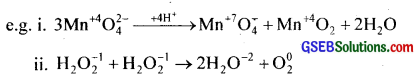Question 22.
Which metal in the first series of transition metals exhibits +1 oxidation state most frequently and why?
Copper has electronic configuration [Ar] 3d104s1. It can easily lose one (4s1) electron to give stable 3d10 configuration.

Question 23.
Calculate the number of unpaired electrons in the following gaseous ions:
Mn3+, Cr3+, V3+, and Ti3+. Which one of these is the most stable in an aqueous solution?
Unpaired electrons Mn3+ = 4, Cr3+ = 3, V3+ = 2, Ti3+ = 1. Most stable Cr3+.

Question 24.
Give examples and suggest reasons for the following features of the transition metal chemistry:

1. A transition metal exhibits the highest oxidation state in oxides and fluorides.
2. The highest oxidation state is exhibited in oxoanions of a metal.

1. Oxygen and fluorine are highly electronegative and small in size. Therefore transition metal exhibits higher oxidation states in oxides and fluorides.
2. Oxygen is a highly electronegative and strong oxidising agent. Hence highest oxidation state is exhibited in oxoanions of a metal.Question 25.
Indicate the steps in the preparation of:

1. K2Cr2O7 from chromite ore.
2. KMnO4 from pyrolusite ore.

1. On heating chromite ore (FeCr2O4) with sodium carbonate in the presence of air gives sodium chromate.
4FeCr2O4 + 8Na2CO3 + 7O2 → 8Na2CrO4 + 2Fe2O3 + 8CO2

The soluble sodium chromate (Na2CrO4) on acidification with sulphuric acid produces sodium dichromate (Na2Cr2O7) which on treatment with potassium chloride gives crystals of potassium dichromate.
2Na2CrO4 + H2SO4 → Na2Cr2O7 + Na2SO4 + H2O
Na2Cr2O7 + 2KCl → 2K2MnO4 + 2H2O

2. Potassium permanganate is prepared from pyrolusite, MnO2. Powdered pyrolusite is fused with KOH or K2CO3. The molten liquid is stirred well in the presence of air when green coloured potassium manganate is formed.
2MnO2 + 4KOH + O2 → 2K2MnO4 + 2H2O

Potassium manganate is extracted with water and oxidised electrolytically between iron electrodes to potassium permanganate.
K2MnO4 ⇄ 2K+ + MnO2-4

At anode:
MnO2-4 → MnO4 + e

At cathode:
2H+ + 2e → H2

The overall reaction is
2K2MnO4 + H2O + O → 2KMnO4 + 2KOH
The purple coloured solution on evaporation gives crystals of KMnO4.

Question 26.
What are alloys? Name an important alloy which contains some of the lanthanoid metals? Mention its uses?
An alloy is a blend of metals prepared by mixing the components. Certain lanthanides are used in the production of mischmetal, an alloy which contains rare earth elements (94 – 95%), iron (5%) and traces of sulphur, carbon, silicon, calcium and aluminum.

The main rare earth elements present in this alloy are cerium (≈ 40%), lanthanum and neodymium (≈ 44%). It is pyrophosphoric and is used in cigarette and gas lighters, flame-throwing tanks, tracer bullets etc. Alloy containing magnesium and about 3% mischmetal is used in jet engine parts. The presence of lanthanides in alloys increases the workability of steel at high temperatures.

Question 27.
What are inner transition elements? Decide which of the following atomic numbers are the atomic numbers of the inner transition elements: 29, 59, 74, 95, 102, 104.
The lanthanides and the actinides constitute the inner transition elements (f – block dements). Atomic numbers of the inner transition elements: 59, 95, 102.Question 28.
Which is the last element in the series of actinoids? Write the electronic configuration of this element. Comment on the possible oxidation state of this element?
Both lanthanide and actinide elements involve the filling of f-orbitais. They show close resemblances in some of their properties, but also differ in many properties.

Similarities:

1. Both exhibit a +3 oxidation state predominantly.
2. In both series, f – orbitals are being progressively filled.
3. Both are electropositive and very reactive.
4. Both exhibit magnetic and spectral properties.
5. Like lanthanide contraction, actinide shows actinide contraction due to the poor shielding effect of 5 f – electrons.
6. The lanthanide ions other than f° (La3+ or Ce4+) and f14 (Yb2+ and Lu3+) show paramagnetic due to the presence of unpaired f electrons. Neodymium shows maximum paramagnetism.

Differences:
The important points of difference between lanthanides and actinides are summed up below.

 Lanthanides Actinides 1. Besides common oxidation state of +3, lanthanides show +2 and +4 states. 1. Besides common oxidation state of +3, actinides show higher oxidation states of+4, +5, +6 and +7. 2. The tendency to form complexes is less 2. They have greater tendency to form complexes. 3. Except promethium, all elements are non-radioactive. 3. All actinides are radioactive. 4. Lanthanide compounds are less basic. 4. Actinide compounds are more basic.

In period 7 after Z = 89, when the 5f and 6d-orbitals have almost the same energy. there are 14 actinides which are formed when the 5f-orbitals are filled successively with electrons. There is no 5f electron in thorium. Occupation of electrons in 5f orbitals starts from Pa.

1. Electronic configuration of Actinide elements: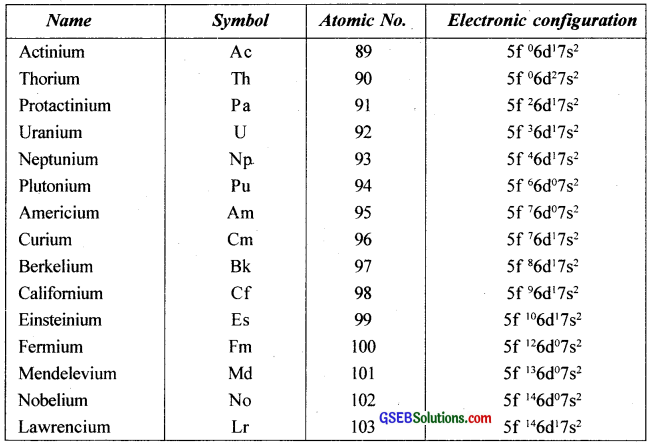2. There is a decrease in the size of atoms and ions with increase in atomic number and is termed as actinide contraction. The decrease is more than in lanthanides because the shielding by 5f is less effective than that by 4f electrons.

Other properties:
Actinides are more reactive than lanthanides. This is partly due to the bigger size. The exposure of 5f orbitals is to such an extent that they get involved in chemical reactions. Hydrochloric acid attacks all metals but most are slightly affected by nitric acid owing to the formation of protective layer; alkalies have no action.

Uses:
Uranium, thorium and neptunium are used as fuel for atomic reactors. Thorium salts are used in making incandescent gas mantles. Uranium salts are used in glass industry, textile industry ceramic industry and in medicines.

3. All these metals are radioactive and therefore, their accessibility for laboratory investigations is limited. The elements beyond uranium (At. No. 92) are all man-made elements and are made by nuclear-chemical methods. The chemistry of actinide elements is more complex in view of their ability to exist in different oxidation states. The common oxidation state of these elements is +3 However. they also exhibit oxidation states of +4, +5 and +6.

This is due to

• The difference between 5f and 7s orbitals is very small and
• 5f orbitals in actinides are more exposed than 4f orbitals in lanthanides and so 5felectrons are also involved in chemical reactions.

4. Chemical reactivity:

Chemical reactivity of the elements:-
Reactivity refers to how likely or vigorously an atom is to react with other substances. The farther to the left and down the periodic chart you go, the easier it is for electrons to be given or taken away, resulting in higher reactivity.Question 29.
Use Hund’s rule to derive the electronic configuration of Ce3+ ion, and calculate its magnetic moment on the basis of ‘spin-only’ formula?
58Ce = [Xe] 4f15d16s2
Ce3+ = [Xe] 4f1
∴ µ = $$\sqrt{n(n+2)}$$ BM = $$\sqrt{1(1+2)}$$ BM = $$\sqrt{3}$$ = 1.73 BM

Question 30.
Name the members of the lanthanoid series which exhibit +4 oxidation states and those which exhibit +2 oxidation states. Try to correlate this type of behaviour with the electronic configurations of these elements?
Lanthanoid with configuration close to f°, (4f°, 4f1, 4f2) or close to 4f7 (4f7 or 4f8) shows +4 oxidation state. +4 state is exhibited by 58Ce, 59Pr, 60Nd, 65Tb, 66Dy Lanthanoid with 5d° 6s2 configuration shows +2 oxidation state. +2 state is exhibited by 60Nd, 62Sm, 63EU, 69Tm, 70Yb.

Question 31.
Compare the chemistry of the actinoids with that of lanthanoids with reference to:

1. Electronic configuration
2. Oxidation states and
3. Chemical reactivity

Both lanthanide and actinide elements involve the filling of f – orbitals. They show close resemblances in some of their properties, but also differ in many properties.

Similarities:

1. Both exhibit +3 oxidation state predominently.
2. In both series, f – orbitals are being progressively filled.
3. Both are electropositive and very reactive.
4. Both exhibit magnetic and spectral properties.
5. Like lanthanide contraction, actinide shows actinide contraction due to the poor shielding effect of 5 f – electrons.
6. The lanthanide ions other than f° (La3+ or Ce4+) and f14 (Yb2+ and Lu3+) show paramagnetism due to the presence of unpaired f electrons. Neodymium shows maximum paramagnetism.

Differences:
The important points of difference between lanthanides and actinides are summed up below.

 Lanthanides Actinides 1. Besides common oxidation state of +3, lanthanides show +2 and +4 states. 1. Besides common oxidation state of +3, actinides show higher oxidation states of+4, +5, +6 and +7. 2. The tendency to form complexes is less 2. They have greater tendency to form complexes. 3. Except promethium, all elements are non-radioactive. 3. All actinides are radioactive. 4. Lanthanide compounds are less basic. 4. Actinide compounds are more basic.

Question 32.
Write the electronic configurations of the elements with the atomic numbers 61, 91, 101, and 109?
61Pm: [Xe] 4f55d°6s2
91Pa: [Rn] 5f26d17s2
101Md: [Rn] 5f136d°7s2
109Mt: [Rn] 5f146d77s2Question 33.
Write down the number of 3d electrons in each of the following ions: Ti2+, V2+, Cr3+, Mn2+, Fe2+; Fe3+, CO2+, Ni2+ and Cu2+. Indicate how would you expect the five 3d orbitals to be occupied for these hydrated ions (octahedral)?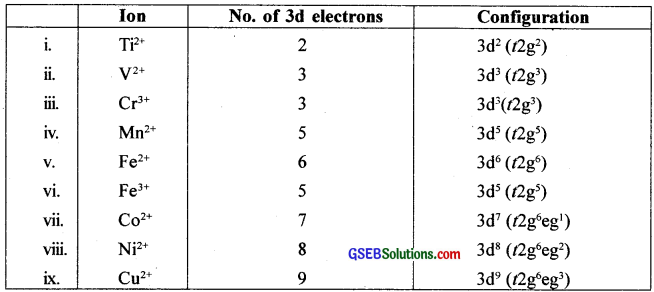Question 34.
What can be inferred from the magnetic moment values of the following complex species?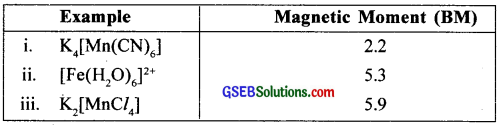Magnetic moment, µ = $$\sqrt{n(n+2)}$$ BM or µ2 = n(n + 2)

1. For K4[Mn(CN)6], µ = 2.2
i.e., n(n + 2) = (2.2)2 ∴n ≈ 1
Since CN is a strong field ligand, the electrons in 3d pair up.
∴ Mn2+ undergoes d2sp3 hybridisation giving inner orbital complex.

2. For [Fe(H2O6)6]2+, µ = 5.3
i.e., n(n + 2) = 5.32
∴ n ≈ 4
Since H2O is a weak field ligand, the 3d electrons do not pair up.
∴ Fe2+ undergoes sp3d2 hybridisation giving an outer orbital complex.

3. For K2[MnCl4], µ = 5.9
i.e., n(n + 2) = 5.92
∴ n ≈ 5
Since Cl is a weak field ligand, the 3d electrons will not pair up.
∴ Mn2+ undergoes sp3 hybridisation giving a tetrahedral complex.

### GSEB Class 12 Chemistry d-and f-Block Elements Additional Important Questions and Answers

Question 1.
The electronic configuration of copper written by two students is given below.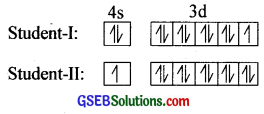2. Copper is considered as a transition metal. Give reason.
3. Zn, Cd and Hg are generally not considered as transition metals. Why?

1. Student-II wrote the correct electronic configuration. 4s13d10 electronic configuration attains extra stability due to the symmetrical arrangement of electrons in 3d orbitals and the maximum value of exchange energy.

2. Copper is regarded as a transition metal though it has completely filled d-orbitals (3d10). This is because, in the most stable oxidation state, Cu2+ ion has partially filled d orbitals (3d9)

3. These elements in their atomic form and in the most common oxidation state of +2 have completely filled d – orbitals. Hence they are generally not considered transition metals.Question 2.
How do atomic radii of transition elements in a given series vary with an increase in atomic number? What is your observation on atomic radii of the 4d and 5d series of transition elements? Discuss the phenomenon involved, if any
The 4d and 5d series of transition elements in each group have the same atomic radii. This is due to lanthanide contraction.
The steady decrease in the size of the atoms or ions from lanthanum to lutetium is known as lanthanide contraction.

As the atomic number increases in the lanthanide series, the new electron goes to the inner 4f orbitals. The shielding effect of a 4f electron is poor. As a result, the attraction of the outer electrons by the nucleus increases and this tends to a decrease in size.

Question 3.
Transition metals are widely used as catalysts in industrial processes?

1. Give any two industrial processes in which d-block elements are used as catalysts.
2. Transition metals exhibit catalytic properties. Why?
3. Why do the transition elements exhibit similarity in properties along the period as well as down the group?

1. (a) Fe is used in the Haber process.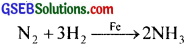(b) Pt in presence of V2O5 is used in the Contact process.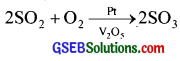2. Due to the presence of unpaired d – orbitals, transition metals show a variable oxidation state. It can easily pass from one state to another and enables the formation of unstable intermediate compounds. Thus, they exhibit catalytic properties.

3. In transition elements, the differentiating electron enters in the (n – 1)d orbitals. Thus the number of electrons in the last energy level remains the same along the period as well as down the group. Thus, transition elements exhibit similarity in properties along the period as well as down the group.Question 4.
Give reason?

1. Zn2+ salts are white while Ni2+ salts are blue in colour.
2. Cr is a hard metal while Hg is a liquid.
3. Mn2+ shows maximum paramagnetism among the bivalent ions of the first transition series.
4. Cu2+ is paramagnetic while Cu+ is diamagnetic.
5. First ionisation energies of 5d elements are higher than those of 3d and 4d elements.

1. Zn2+ has completely filled d orbitals (3d10) while Ni2+ has incompletely filled d orbitals. Thus d-d transition of electrons is possible in Ni2+ ion and it is coloured.

2. Due to the presence of five unpaired d electrons, (3d54s1), the interatomic bonding in chromium is very strong and hence it is a hard metal. But in mercury the atoms have fully filled d – orbitals (5d106s2) and the interatomic bonding in them is very weak. Hence mercury is a liquid.

3. Paramagnetism arises due to the presence of unpaired d – electrons. In the first transition series Mn2+ ion has a maximum number of unpaired electrons (3d5). Hence the maximum paramagnetism is observed in the case of Mn2+ ions.

4. Cu2+ has 3d9 electronic configuration and Cu+ has 3d10 electronic configuration. Due to the presence of an unpaired d – electron, Cu2+ shows paramagnetic properties and due to the absence of unpaired d – electrons. Cu+ shows diamagnetic properties.

5. Due to the poor shielding effect of 4f electrons in 5d transition elements, the outermost electrons are strongly attracted by the nucleus. Therefore the first ionization energies of 5d elements are higher than those of 3d and 4d elements.Question 5.
The following is a part of ‘d’ – block of the periodic table.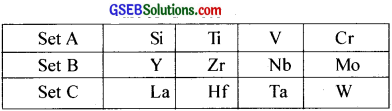1. Compare the atomic size of elements of set A & B and set B & C of same group?
2. Name the phenomenon behind the relation between elements of set B & C?
3. Give reason for the phenomenon?

1. Atomic size of set B elements is greater than that of set A elements because new shell is added. But the atomic size of set B elements is comparable with that of set C elements.
2. Phenomenon behind the relation between elements of Set B and C is lanthanide contraction.
3. Lanthanide contraction is due to the poor shielding effect of very diffused ‘f’ orbitals.

Question 6.
The f-block elements are known as lanthanides and actinides.
a. Why they have similar physical and chemical properties?
b. Compare the similarities and differences between lanthanides and actinides?
c. Give the important uses of them.
a. Both lanthanides and actinides involve the filling of (n – 2) f orbitals. Also they have very similar atomic and ionic radii. Hence their chemical and physical properties are alike.

b. Similarities

• Both exhibit a +3 oxidation state predominantly.
• In both series, f – orbitals are being progressively filled.
• Both are electropositive and very reactive.
• Both exhibit magnetic and spectral properties.
• Like lanthanide contraction, actinide shows actinide contraction due to the poor shielding ef- feet of 5 f – electrons.

Differences
The important points of difference between lanthanides and actinides are summed up below.

 Lanthanides Actinides 1. Besides common oxidation state of +3, lanthanides show +2 and +4 states. 1. Besides common oxidation state of +3, actinides show higher oxidation states of+4, +5, +6 and +7. 2. The tendency to form complexes is less 2. They have greater tendency to form complexes. 3. Except promethium, all elements are non-radioactive. 3. All actinides are radioactive. 4. Lanthanide compounds are less basic. 4. Actinide compounds are more basic.

c.

• Lanthanides are used in the production of mischmetal (alloy of rare earth).
• Lanthanide oxides are used as phosphorus in colour TV.
• Uranium and thorium are used as fuel in atomic reactors.
• Thorium is used for making incandescent gas mantles.Question 7.
Ramu found that in the qualitative analysis of Cu2+ ions when NH3 is added to it, a blue coloured solution is obtained?

1. Identify the compound formed.
2. Explain the chemistry of the reaction.
3. Transition metals readily form complexes. Why?
4. [Ti(H2O)6]3+ is coloured while [Sc(H2O)6]3+ is colourless. Explain?
5. Why Zr and Hf exhibit similar properties?